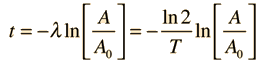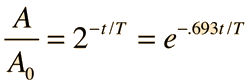The radioactive half-life for a given radioisotope is a measure of the tendency of the nucleus to "decay" or "disintegrate" and as such is based purely upon that probability. Alternatively, this information can be expressed in terms of the decay constant λ. The number of atoms, the mass of the substance, and the level of activity all follow the same exponential decay form. The calculation below is stated in terms of the amount of the substance remaining, but can be applied to intensity of radiation or any other property proportional to it.

If the radioactive half-life of an isotope is
T = x10^ s = min = hr
T = yr = x10^ yr

and amount A0 is present at time t=0 and amount A remains at time t, then= x10^ s = min
t = hr = yr = x10^ yr

the fraction remaining will be given by= = x10^

If the beginning amount was Ci* then the amount remaining is Ci

This calculation is designed for exploration. It is presumed that you will enter a half-life for a particular radioisotope. Then if you enter a time, then it will calculate the fraction remaining at that time. If you enter a fraction or amount remaining, it will calculate the time required (or age) required to achieve that fraction.

*The amount is listed in Ci (Curies), but the mass, intensity of radiation, number of counts or other ways of characterizing the radioisotpe will decrease by the same ratio, so the calculation can be used for any of those quantities.

 Graph of decay Half-life discussion
Index

 HyperPhysics***** Nuclear R Nave
Go Back